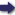(London :  Kegan Paul, Trench, Trübner & Co.,  1910.)

 Tools

## Search this bookPrev Page 31 Next``` CHAPTER LII. 31 magupta, as according to him a caturyuga, is simply one- page 218. thousandth of a kcdpa,. We should only have to shorten the above-mentioned numbers by three ciphers, and in every other respect get the same results. Therefore we shall now give this computation according to the theory of Pulisa, which, though applying to the caturyuga, is similar to the method of computation used for a kalpa. According to Pulisa, in the moment of the beginning of the gauge-year, there have elapsed of the years of the ccduryuga 3,244,132, which are equal to 1,167,887,520 solar days. If we multiply the number of months which corresponds to this number of days with the number of the adhimdsa months of a caturyuga or a corresponding multiplicator, and divide the product by the number of the solar months of a ccduryuga, or a corresponding divisor, we get as the number of adhi¬ mdsa maxAhs, 1,196,52511^^-^-. Further, the past 3,244,132 years of the ccduryuga are 1,203,783,270 lunar days. Multiplying them by the number of the "dnardtra days of a caturyuga, and dividing the product by the lunar days of a caturyuga, we get as the number of Unardtra days i8,835,700-2^'^^f^-^^V- Accordingly, the civil days which have elapsed since the beginning of the caturyuga are 1,184,947,570, and this it was which we wanted to find. We shall here communicate a passage from the a similar Pulisa-siddhdnta, describing a similar method of com- computation putation, for the purpose of rendering the whole subject ^Q^puUm- 1 j_j_i •Ti"j_i 1 ~\ n • 'j^ji siddhdnta. clearer to the mmd of the reader, and nxmg it there more thoroughly. Pulisa says: " We first mark the kalpas which have elapsed of the life of Brahman before the present kalpa, i.e. 6068. We multiply this number by the number of the caturyugas of a kalpa, i.e. 1008. Thus we get the product 6,116,544. This number we multiply by the number of the yugas of a caturyuga, i.e. 4, and get the product 24,466, i y6. This number we multiply by the number of years of a yugco, ```Prev Page 31 Next